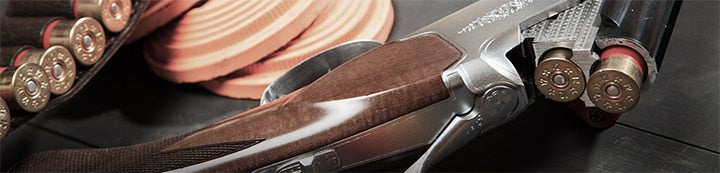Status
Not open for further replies.
1 - 11 of 11 Posts

#### pullbangloss

·
##### Registered
Joined
·
1,831 Posts
Discussion Starter
Hey All,

My current formula reads =IF(B2=33,M2,""). What I want is for the cell to equal M2 if either B2=33 or B2=43. Any help would be appreciated.

Matt Ski

#### paul.QTGC

·
##### Registered
Joined
·
9 Posts
try this
=IF(AND(B2<36,B2>32),M2,"")

#### paul.QTGC

·
##### Registered
Joined
·
9 Posts
=IF(OR(B2=33,B2=43),M2,"")

#### texasaggie2000

·
##### Registered
Joined
·
63 Posts
If you want M2 to equal the discrete numbers of 33 or 43, try this:

=if(b2=33,m2,if(b2=43,m2,""))

·
Joined
·
6,204 Posts
try a calculator

#### zzt

·
##### Registered
Joined
·
4,113 Posts
Paul's second example is the correct formula, although texasaggie's will also return the value of M2 if true.

#### 320090T

·
##### Registered
Joined
·
4,021 Posts
Now, one of you guys explain all that in regular terms, please, thanks.

#### jhoward

·
##### Registered
Joined
·
829 Posts
=IF(OR(B2=33,B2=43),M2,"")

=If(expression, if true, if false)

Basically, the equation evaluates the 'expression' to determine if it is true or false. In this case is "OR(B2=33,B2=43)" true or false? I'll go over the 'OR' statement in a minute. If the 'expression' is true, then Excel will use the first value given, in this case the value in cell M2. If the 'expression' is false, then Excel will use the second value of "".

OR(B2=33,B2=43)

If the value in cell B2 = 33 OR 43 then the expression returns a value of 'true', if the value in cell B2 is something other than 33 or 43 then the expression returns a value of 'false'.

=IF(OR(B2=33,B2=43),M2,"")

If the value in cell B2=33 or 43 then use the value in cell M2 in this cell, otherwise leave this cell blank.

#### 320090T

·
##### Registered
Joined
·
4,021 Posts
And what might this be used for?

#### pullbangloss

·
##### Registered
Joined
·
1,831 Posts
Discussion Starter
Thanks to all for your help. I knew there had to be some Excel guru's out there somewhere.

Mitch,
Thanks for the congrats. It wasn't pretty but we got the job done! I learned the secret to winning calcuttas...have a good partner!

Thanks again to EVERYONE!

Matt Ski

#### wolfram

·
##### Registered
Joined
·
6,754 Posts
Try =if(b2=33,m2,if(b2=43,m2),""). Nested if statement. If you get into more conditions that this you may want to use the lookup function and a table. Its alot easier to modify and expand.

1 - 11 of 11 Posts
Status
Not open for further replies.